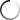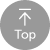B1 中級 10

 Okay, So what I have here is I've taken this transistor circuit that we just built and built another copy of it just up here and just sort of connected them in parallel here. So So, basically, I have two independent copies of this transistor circuits. So just just to clarify what's going on? Um, if we look at the bottom circuit, we have our power coming in. Going through are led through the resistor, uh, into the, um, the emitter of the two. This is the collector of the transistor. And then, of course, our base is connected to our signal that's coming in from our little mystery device. And then, uh, the current is flowing out of the emitter back to, uh, back to ground over here and then on top, I have the exact same thing. I just connected the positive power supply up to the top here, and so that's going through. This led through this resistor, Um and then this transistor is going from the collector to the emitter and then back down. I just connected the ground from from the top here to the bottom. So the positive voltage and the ground or just connected so that these are both getting power. And then I've also connected the signal to both bases. That's what this you little yellow jumper is is I've connected the signal that's coming in from our little mystery device to both bases. And so now we see that this transistor switching this led this transistors. Now switching this led. And the whole reason I did that is just so that I can make some modifications to one of these circuits, um, and keep the other circuit just as it was, because I hopefully we understand what's going on with this circuit down here. And I could make some modifications to this and just sort of compare what's going on between the two. So I'm gonna make a modification, and basically the modification I'm gonna make is I'm just gonna remove the led from the top circuits. I'm gonna take my power, and instead of going to the led, I'm gonna go to the resistor and I'm gonna remove the led from from the circuit altogether. So now the power for the top one is coming in here. The voltage positive altar just connected to the resistor, the resistor through the transistor and then to ground on. There's no led. So this is not maybe not quite as interesting because there's nothing to look at. Um, but the transistor is should be still switching because it's still connected to our signal. So let's take a look at a schematic of what we've got. So this is the schematic. And, um, basically, I have the positive five volt supply here, going through the resistor through the transistor to ground, and there's no L e D. Now, um, and then I still have our little mystery device sending its signal, um, and creating the current, that switching on and off from the base to the emitter like this, and so that base current is still switching on and off. And so, as a result, the collector current should still be switching on and off. It's just going through the resistor to ground, and there's no led. So it's maybe not as interesting to look at. But let's think about what are some different voltages that air that air along here. So if we look at the very top here, we look right here, um, at the voltage that's there, and when I say voltage at a point. Of course you can't. You can't talk about voltage. It appoint voltage has to be between two points, so I'm always gonna be referencing to ground. So the voltage between here and ground is is five volt is always five volts, right? This is just this point here. If we look at that particular point, it's always connected to five volts, so that's always five volts. Conversely, if I come down here and I look at the voltage at this point reference to ground well, it's connected directly to ground, so there's zero voltage there. But what about this point right here? What is the voltage here? Both when this current is on, when the transistor is on and when the transistor is off? So let's let's consider the transistor off right now. So if the transistor is off, then the voltage here is going to be five volts. Because again, we can kind of imagine that the transistor is not there all together. So there's no there's nothing. There's no, uh, conductivity through this transistor, and so this point has a resistor, but it's connected to five volts, so there's five volts at this point. um, with reference to ground because the sort of if you think of the path of least resistance for electrons, they're gonna go through this resistor to five volts. There's no other place for them to go because the transistor is off. Now, when current is flowing here, the transistor is on. Then this point here is gonna have what kind of voltage? Well, right now, if the transistor is on the path of least resistance for electrons, Uh, that that come in here is going to be to ground. And so the voltage, if we measure the voltage here, will actually be zero, because the path of least resistance is to ground not through this resistor to five volts. So this is kind of interesting, because just just to clarify here, when the transistor is off this point, let's call it, you know, point. Ah, I know. It's called point A. Right here, A is equal to five volts, right? The transistors off. So this is the potential here is is connected through this resistor to five volts. But when the transistor is on and in saturation, which means that the transistor is basically completely on, it's not. It's not restricting current at all, which means we have enough current here to create that saturation. So when the transistor is on between, we have current flowing here. Then the voltage at a is equal to zero volts because again there the path of least resistance is going to be from a to ground. So in effect, a is connected directly to ground on DSO. The voltage today is is gonna be zero with reference to ground. So this seems maybe a little bit backwards Then then what? You might think when the transistor is off. We have five volts here. When the transistor is on. We have zero volts here. So maybe we could connect and led to this point A. Maybe we could connect in, led here and actually kind of see this sort of backwards, if you will. Voltage. Um, so let's let's let's hook up in led right here and we'll connect it to point a there. And then, of course, we'll connect this side down to ground. So essentially we're connecting. The led from from the collector to the emitter of of the transistor were just connecting the led across. So let's do that in the circuit here, So I have my led, and I'm just gonna connect it from just across the collector to the emitter like that. And sure enough, we see the led is on when our signal, when are when our base current is off. And it's off when our base current is on. And we could see that, of course, because this bottom circuit, we haven't modified it all. And so you know, this led is on when the bass current is on and this current ability is off when the bass current is on. And so essentially, we're seeing the the opposite of what of what our signal is. So our signal, remember, is turning on and off the same time as this led. But this led up here is giving us the opposite of that. So that's very interesting, because maybe there's Maybe there's some application to that, but just to clarify before before I end this video just to clarify, kind of to give a little bit more intuition about what's going on, you can imagine, um, a circuit here with a battery so we'll have a battery here, and we'll connect the battery through resistor to a switch. Actually, we won't even put a switch here. We'll put in Led. We'll have an led and then back to the battery. And so this is our positive terminal courses. They're negative terminal. So in this case, the led would be on no surprises there. But if I put a switch over here in parallel with our led and so I have a switch right here like this when the switch is off, In other words, the switches open. Then the led is on, right. The circuits gonna flow this way. But if I turn the switch on that is closing the switch, then I actually create a short circuit around the led and the led will go off. The current will flow through the resistor, threw the switch, which offers less resistance than the led, and then and then back around. And so in this case, you can see the switch being on turns. The led off in the switch being off turned the led on. And that's exactly what's happening with the transistor. Because look at the transistor. We have the transistor now in parallel with with the led Ah, And so when the transistor is off current flows through the led when the transistor is on current flows through the transistor basically a short circuit through the transistor bypassing the led in the led turns off. So hopefully that that was interesting. I think it's interesting because it gives us the ability now to basically take a signal like this and basically inverted and get the opposite of that signal by using a transistor.

# Inverting the signal with a transistor | Digital electronics (9 of 10)

10

#### 影片學習單字重點#### 影片討論1. ## 1. 單字查詢

在字幕上選取單字即可即時查詢單字喔！

2. ## 2. 單句重複播放

可重複聽取一句單句，加強聽力！

3. ## 3. 使用快速鍵

使用影片快速鍵，讓學習更有效率！

4. ## 4. 關閉語言字幕

進階版練習可關閉字幕純聽英文哦！

5. ## 5. 內嵌播放器

可以將英文字幕學習播放器內嵌到部落格等地方喔

6. ## 6. 展開播放器

可隱藏右方全文及字典欄位，觀看影片更舒適！

1. ## 英文聽力測驗

挑戰字幕英文聽力測驗！

1. 點擊展開筆記本讓你看的更舒服

1. UrbanDictionary 俚語字典整合查詢。一般字典查詢不到你滿意的解譯，不妨使用「俚語字典」，或許會讓你有滿意的答案喔